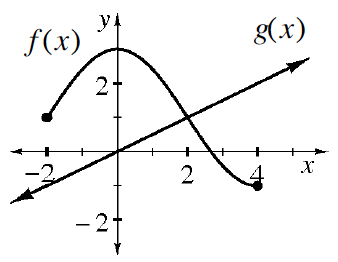### Home > CCA > Chapter Ch11 > Lesson 11.1.1 > Problem11-12

11-12.

Examine the graphs of relations $f(x)$ and $g(x)$ below. Use the graph to approximate the values below (if possible).1. $f(0)$

When $x$ is $0$ in the relation $f(x)$, what is $y$?

$3$

1. $g(4)$

When $x$ is $4$ in the relation $g(x)$, what is $y$?

$2$

1. $f(5)$

Is there a $y$-value for $f(x)$ when $x$ is $5$?

1. $g(0)$

See the hint for part (b).

1. $g(2)$

See the hint for part (b).

1. $f(2)$

See the hint for part (a).